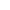Maths | CBSE Board | Grade 12 | 2013
Q. If matrix A= and A2=KA, then write the value of K.
View SolutionQ. Write the principle value of tan1(3)cot1(3).
View SolutionView SolutionQ. Consider f:R+[4, ] given by f(x)=x2+4. Show
that f is invertible with the inverse f1 of f given by f1(y)=y4 where R+ is the set of all non-negative real numbers.
View SolutionQ. Find the area of the greatest rectangle that can be inscribed in a ellipes x2a2+y2b2=1.
View SolutionQ. Find the area of the region bounded by the parabola y=x2 and y=|x|.View SolutionQ. If yx=eyx, prove that dydx=(1+logy)2logy.
View SolutionQ. A manufacturer considers that men and women workers are equally efficient and so he pays them at the same rate. He has 30 workers (male and female) and 17 units capital; which he uses to produce two types of goods A and B. To produce one unit of A, 2 workers and 3 units of capital are required while 3 workers and 1 unit of capital is required to produce one unit of B. If A and B are priced at Rs. 100 and Rs. 120 per unit respectively, how should he use his resources to maximise the total revenue? Form the LPP and solve graphically.
Do you agree with this view of the manufacturer that men and women workers are equally efficient and so should be paid at the same rate?
1. 1290
2. 1260
3. 1130
4. 3421
View SolutionQ. The probabilities of two students A and B coming to the school in time are 37 and 57 respectively. Assuming that the events' A coming in time' and 'B coming in time' are independent. Find the probability of only one of them coming to the school in time. Write atleast one advantages of coming to school in time.
View SolutionQ. If matrix A= and A2=pA, then write the value of p.
View SolutionQ. Find the Cartesian equation of the line which passes through the point (-2, 4, -5) and is parallel to the line x+33=4y5=z+86.
View SolutionQ. The money to be spent for the walfare of the employees of a firm is proportional to the rate of change of its total revenue (marginal revenue). If the total revenue (in rupees) received from the sales of x units of a product is given by R(x)=3x2+36x+5, find the marginal revenue, when x=5, and write which value does the question indicates.
View SolutionQ. Find the length of the perpendicular drawn from the origin to the plane 2x3y+6z+21=0.
View SolutionQ. Find the particular solution of the differential equation (tan1yx)dy=(1+y2)dx, given that when x=0, y=0.
View SolutionQ. Write the differential equation representing the family of curves y=mx, where m is an arbitrary constant.
View SolutionQ. Evaluate : 2π011+esinx
View SolutionQ. Evaluate dxx(x5+3).
View SolutionQ. For what value of x, is the matrix A=011103x30 a skew symmetric matrix?
View SolutionQ. Using properties of determinants prove the following:
∣ ∣xx+yx+2yx+2yxx+yx+yx+2yx∣ ∣=9y2(x+y).
View SolutionQ. Show that : tan(12sin134)=473.
View SolutionQ. In a hockey match, both teams A and B scored same number of goals up to the end of the game, so to decide the winner, the referee asked both the captains to throw a die alternately and decided that the team, whose captain gets a six first, will be declared the winner. If the captain of team A was asked to start, find their respective probabilities of winning the match and state whether the decision of the referee was fair or not.
View SolutionQ. Write the value of tan1[2sin(2cos132)].
View SolutionQ. Show that the lines
r=3^i+2^j4^k+λ(^i+2^j+2^k)
r=5^i2^j+μ(3^i+2^j+6^k)
are intersecting. Hence find their point of intersection.
View SolutionQ. Differentiate the following with respect to x: sin1(2x+13x1+(36)x).
View SolutionQ. If Aij is the cofactor of the element aij of the determinant 235604157 then write the value of a32.A32.
View SolutionQ. Find the equation of the plane passing through the line of intersection of the planes r.(^i+3^j)6=0 and r.(3^i^j4^k)=0 whose perpendicular distance from origin is unity.
View SolutionQ. P and Q are two points with position vectors 3a2b and a+b respectively. Write the position vector of a point R which divides the line segment PQ in the ratio 2:1 externally.
View SolutionQ. If a=^i^j+7^k and b=5^i^j+λ^k, then find the value of λ, so that a+b and ab are perpendicular vectors.
View SolutionQ. Find the value of k, for which f(x)=⎪ ⎪ ⎪⎪ ⎪ ⎪1+kx1kxxif1x<02x+1x1if0x<1⎪ ⎪ ⎪⎪ ⎪ ⎪ is continuous at x=0.
View SolutionQ. Find |x| if for a unit vector a, (xa).(x+a)=15.
View Solution